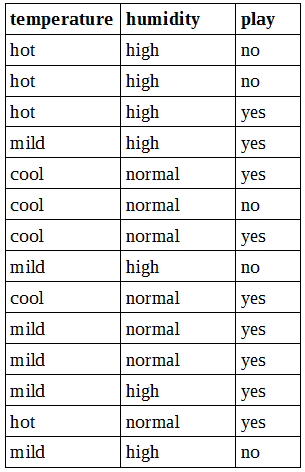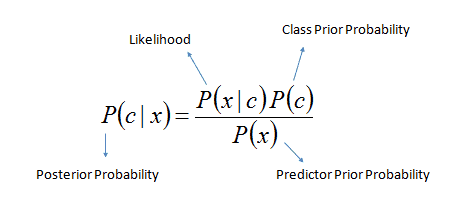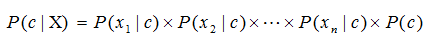# Simple explanation of Naive Bayes classifier

Probably you’ve heard about Naive Bayes classifier and likely used in some GUI based classifiers like WEKA package. This is a number one algorithm used to see the initial results of classification. Sometimes surprisingly it outperforms the other models with speed, accuracy and simplicity.Lets see how this algorithm looks and what does it do.

As you may know algorithm works on Bayes theorem of probability which allows to predict the class of unknown data set. Hoe you are comfortable with probability math – at least some basics.

## Naive Bayes basics

Naive Bayes algorithm relies on assumption that features are independent to each other. For instance if you take features like temperature, humidity and wind speed to predict the rain you would assume that all those three features independently contribute to probability of upcoming rain. Even if these features have some relation we would naively tell that they aren’t. This is one of reasons why algorithm is called ‘Naive’.

Naive Bayes algorithm is very handy on very large data sets, because it’s fast, simple and accurate when compared to other classification algorithms.

Lets dig in to some math to understand the basics.

Bayes algorithm is based on posterior probability that combines previous experience and likelihood of event. To understand all of that let us look at a simple example. Bellow you can see whether data set with two features (temperature, humidity) and class (play). We will want to build a Naive Bayes predictor which would tell if on current conditions weather is suitable for playing golf or not.We would like to know if temperature cool, along with high humidity is suitable for playing golf.

According to Bayes theorem we need to calculate posterior probabilityor simply we calculate in expanded form:We need to calculate it for each class and then compare the results to find which gives the higher score.

Lets get to our data and go through step by step.

Since we want to classify temperature cool and humidity high we need to find following probabilities:

P(cool|yes), P(high|yes),

P(cool|no), P(high|no),

P(yes), P(no),

optionally we might want to calculate P(cool) and P(high), as you will see this isn’t necessary for basic classification.

P(cool|yes) = (likelihood of cool given yes) / (total number of yes) = 3 / 9 = 1/3;

P(high|yes) = (likelihood of high given yes) / (total number of yes) = 3 / 9 = 1/3;

P(cool|no) = (likelihood of cool given no) / (total number of no) = 1/5;

P(high|no) = (likelihood of high given no) / (total number of no) = 4/5;

P(yes) = (number of yes) / (total number of play) = 9/14;

P(no) = (number of no) / (total number of play) = 5/14;

also lets calculate P(cool) and P(high):

P(cool) = (number of cool) / (total number of temperature) = 4/14;

P(high) = (number of high) / (total number of humidity) = 7/14 = 1/2;

To get answer we then calculate two posterior probabilities and compare results:$P(yes|cool, high) = \frac{P(cool|yes) \cdot P(high|yes) \cdot P(yes)}{P(cool)\cdot P(high)))} = \frac{1/3\cdot 1/3\cdot 9/14}{4/14\cdot 1/2} = 0.5$$P(no|cool, high) = \frac{P(cool|no) \cdot P(high|no) \cdot P(no)}{P(cool)\cdot P(high)))} = \frac{1/5\cdot 4/5\cdot 5/14}{4/14\cdot 1/2} = 0.4$

As we can see that 0.5 > 0.4 we can predict that temperature cool and high humidity is suitable to play golf.

Once you get used to it, Naive Bayes become really easy algorithm to implement. Due to its simplicity, it can be implemented in real time predictors, works well with multiple classes that leads to spam filtering, text classification.